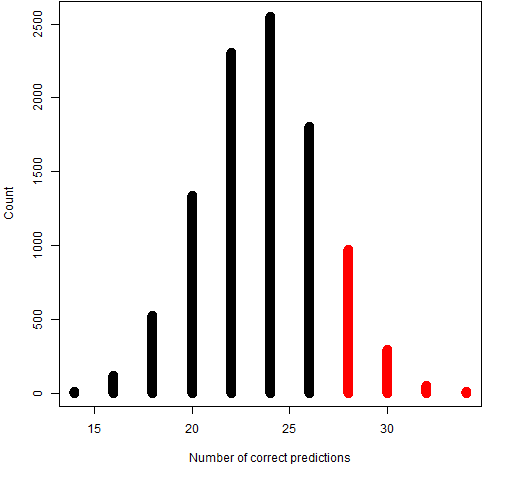# Posts Tagged ‘ P-value ’

## The US market will absolutely positively definitely go up in 2012

February 6, 2012
ByThe Super Bowl tells us so. The Super Bowl Indicator The championship of American football decides the direction of the US stock market for  the year.  If a “National” team wins, the market goes up; if an “American” team wins, the market goes down. Yesterday the Giants, a National team, beat the Patriots. The birth … Continue reading...

## Truly random [again]

December 9, 2010
By“The measurement outputs contain at the 99% confidence level 42 new random bits. This is a much stronger statement than passing or not passing statistical tests, which merely indicate that no obvious non-random patterns are present.” arXiv:0911.3427 As often, I bought La Recherche in the station newsagent for the wrong reason! The cover of the## Random sudokus [p-values]

May 21, 2010
ByI reran the program checking the distribution of the digits over 9 “diagonals” (obtained by acceptable permutations of rows and column) and this test again results in mostly small p-values. Over a million iterations, and the nine (dependent) diagonals, four p-values were below 0.01, three were below 0.1, and two were above (0.21 and 0.42).## The distribution of rho…

March 21, 2010
ByThere was a post here about obtaining non-standard p-values for testing the correlation coefficient. The R-library SuppDists deals with this problem efficiently. library(SuppDists) plot(function(x)dPearson(x,N=23,rho=0.7),-1,1,ylim=c(0,10),ylab="density") plot(function(x)dPearson(x,N=23,rho=0),-1,1,add=TRUE,col="steelblue") plot(function(x)dPearson(x,N=23,rho=-.2),-1,1,add=TRUE,col="green") plot(function(x)dPearson(x,N=23,rho=.9),-1,1,add=TRUE,col="red");grid() legend("topleft", col=c("black","steelblue","red","green"),lty=1, legend=c("rho=0.7","rho=0","rho=-.2","rho=.9"))</pre> This is how it looks like, Now, let’s construct a table of critical values for some arbitrary or not significance levels. q=c(.025,.05,.075,.1,.15,.2) xtabs(qPearson(p=q, N=23, rho

## Contingency Tables – Fisher’s Exact Test

March 6, 2010
By

A contingency table is used in statistics to provide a tabular summary of categorical data and the cells in the table are the number of occassions that a particular combination of variables occur together in a set of data. The relationship between variables in a contingency table are often investigated using Chi-squared tests. The simplest contingency